Journal of Astronomy and Space Sciences
The Korean Space Science Society
Research Paper

# Recovery of Asteroids from Observations of Too-Short Arcs by Triangulating Their Admissible Regions

Grupo de Investigación en Astroingeniería Alfa Orión, Observatorio Astronómico, Universidad Tecnológica de Pereira, Complejo Educativo La Julita, 660003 Pereira, Colombia
Corresponding Author Tel: +57-3163873180, E-mail: daesmo95@utp.edu.co

Received: Oct 3, 2021; Revised: May 28, 2021; Accepted: May 28, 2021

## Abstract

The data set collected during the night of the discovery of a minor body constitutes a too-short arc (TSA), resulting in failure of the differential correction procedure. This makes it necessary to recover the object during subsequent nights to gather more observations that will allow a preliminary orbit to be calculated. In this work, we present a recovery technique based on sampling the admissible region (AdRe) by the constrained Delaunay triangulation. We construct the AdRe in its topocentric and geocentric variants, using logarithmic and exponential metrics, for the following near-Earth-asteroids: (3122) Florence, (3200) Phaethon, 2003 GW, (1864) Daedalus, 2003 BH84 and 1977 QQ5; and the main-belt asteroids: (1738) Oosterhoff, (4690) Strasbourg, (555) Norma, 2006 SO375, 2003 GE55 and (32811) Apisaon. Using our sampling technique, we established the ephemeris region for these objects, using intervals of observation from 25 minutes up to 2 hours, with propagation times from 1 up to 47 days. All these objects were recoverable in a field of vision of 95’ × 72’, except for (3122) Florence and (3200) Phaethon, since they were observed during their closest approach to the Earth. In the case of 2006 SO375, we performed an additional test with only two observations separated by 2 minutes, achieving a recovery of up to 28 days after its discovery, which demonstrates the potential of our technique.

Keywords: admissible region; asteroids; astrometry; ephemerides

## 1. INTRODUCTION

Orbital dynamics of minor bodies of the solar system is a current area of interest in astronomy, especially when these are newly discovered objects, i.e., there are no previous records. Different observatories around the world report the finding of these objects on a daily basis, but due to the short interval of observation (too-short arcs, TSA), the astrometric data collected are not sufficient to establish a preliminary orbit (Gronchi 2004). This is because the classic methods of initial orbit determination fail in this type of case (Milani & Knežević 2005;Espitia et al. 2020).

In order to establish a preliminary orbit for new objects, it is necessary to ensure their re-observation following the nights after the discovery. This task requires anticipating their predicted location in the celestial sphere, a procedure known as recovery (Milani 2001;Milani & Gronchi 2009). The utility available online known as New Object Ephemerides (http://www.minorplanetcenter.net/iau/MPEph/ NewObjEphems.html) is a service offered by the Minor Planet Center (MPC) for the follow-up of new objects. However, this service is based on ephemeris calculation via the orbit-fitting procedure by Väisälä (Gwyn et al. 2012), which is a classic method that provides acceptable results for main-belt asteroids (MBAs) but not for near-Earth asteroids (NEAs; http://www.cadc-ccda.hia-iha.nrc-cnrc. gc.ca/en/ssois/documentation.html#ephem). In addition, this fitting applies the approximation of assuming that the object is observed at its perihelion (Väisälä 1939;Kristensen 2006).

In contrast to classical methods, Milani et al. (2004) use the concept of the admissible region (AdRe) to delimit the location region of an asteroid seen from Earth, followed by triangulation sampling within this region to anticipate the follow-up. While in the literature, there are different applications of this technique in the study of space (Tommei et al. 2007;Maruskin et al. 2009;Farnocchia et al. 2010;DeMars et al. 2012), and in the study of Earth impactors (Valk & Lemaitre 2006;Spoto et al. 2018), the only examples of the method of Milani et al. (2004) being applied for follow-up purposes are NEA 2003 BH84 (Milani et al. 2004) and the centaur (60558) Echeclus (Farnocchia et al. 2015). Given the above, in Espitia Mosquera & Quintero Salazar (2019), we extend this sample by applying the method to delimit the region of space in which a set of 6 asteroids (3 NEA, 2 MBA, and 1 Hilda) were at a given date.

In this article, we present an AdRe sampling method based on the constrained Delaunay triangulation, which can establish a region of possible orbits of a minor body determined from a TSA observation. This set of orbits can determine the search area of the object in the celestial sphere for follow-up purposes. Our technique does not require mesh smoothing, which reduces the computational cost.

In addition, we extend the application sample of the technique of Milani et al. (2004) by determining the AdRe and applying our triangulation in the follow-up of a set of 12 asteroids (6 NEA, 2 MBA, 1 Hungaria, 2 Hilda, and 1 Jupiter trojan).

Based on the results obtained in this work, we discuss the capabilities and limitations of the method.

We implement our follow-up technique in a web service available at http://observatorioenlinea.utp.edu. co/recoveryservice/. The source code of the algorithm is available in that same link under an open-source license.

## 2. MATERIALS AND METHODS

According to Milani et al. (2004), based on an attribute (Milani 2001) given by the expression (1), the AdRe of an object is defined as the set of all possible ($\rho ,\stackrel{˙}{\rho }$) that satisfy the following conditions:

• I. The object belongs to the solar system and is not a longorbital- period celestial body. This implies considering elliptical orbits with heliocentric energies E less than –k2/2amax, with amax = 100 au and k = 0.01720209895 (Gaussian gravitational constant).

• II. The object is not immersed in the Earth’s gravitational field; that is, it has a geocentric energy E ≥ 0 while it is within the Earth’s radius of influence (RSI = 0.010044 au).

$A=\left(\alpha ,\delta ,\stackrel{˙}{\alpha },\stackrel{˙}{\delta }\right)\in \left[-\pi ,\pi \right]×\left(-\frac{\pi }{2},\frac{\pi }{2}\right)×{ℝ}^{2}$
(1)

Condition (1) establishes the upper limit of the AdRe, which can have at most two connected components, in the extreme case in which asteroids with a perihelion greater than 28 au are studied (Spoto et al. 2018). Regarding the lower limit, according to condition (2), it is given by the curve of geocentric energy equal to zero (if 0 < ρ < RSI), or by a straight-line segment ρ = RSI and two arcs corresponding to the geocentric energy. It is even possible to constrain the lower limit further by ignoring the orbits belonging to meteoroids that are too small to be considered sources of meteorites. This is achieved by invoking the condition HHmax (Spoto et al. 2018), with Hmax = 34.5, corresponding to the threshold for meteors (Milani et al. 2004).

In Espitia Mosquera & Quintero Salazar (2019), we determine the AdRe's of a sample of 6 asteroids from the sets of observations that constitute TSAs, using the geocentric and topocentric variants and the logarithmic and exponential metrics. We find that the topocentric variant considerably reduces the search area of the AdRe's since it involves additional constraints, such as the exclusion of meteors. Furthermore, we find that the AdRe's that were generated from a topocentric variant have simpler geometries compared with their geocentric counterparts. Regarding the metrics, we conclude that the logarithmic metric is adequate for analyzing the regions near the lower limit of the AdRe because this scale allows us to see the characteristics at the values in the distance near to the observer, whereas the exponential metric is adequate for the regions near the upper limit. The AdRe's obtained not only excluded those bodies dominated by Earth’s gravity but also considerably reduced the search area, thus optimizing the subsequent sampling from a triangulation.

## 3. SAMPLING OF THE ADRE

The diagram shown in Fig. 1 summarizes the triangulation and follow-up process that we implement in this work. First, we sampled the AdRe boundary through a sampling algorithm for a rectifiable curve (blue block) proposed in Milani et al. (2004). Then, we introduced a sampling strategy for the interior of the AdRe based on the constrained Delaunay triangulation (purple block). Next, we propagated all possible orbits to a later date (red block). Finally, we calculated the ephemerides for each of the propagated orbits; that is, we found the region of location of the body under study in the celestial sphere for a specific date (yellow block).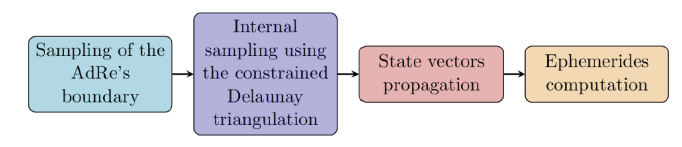Fig. 1. General scheme of the recovery procedure.
3.1 Sampling of the AdRe Boundary

According to Milani et al. (2004), the AdRe has an upper limit given by the arcs of the curve E(ρ,$\stackrel{˙}{\rho }$ ) = 0 or the curve E(ρ,$\stackrel{˙}{\rho }$ ) = –k2/2amax (symmetric with respect to the line $\stackrel{˙}{\rho }$ = –c1/2). In addition, the lower limit is given by the arcs of the curve E⊕(ρ,$\stackrel{˙}{\rho }$ ) = 0 (symmetric with respect to $\stackrel{˙}{\rho }$ = 0) and the segments of the lines ρ = ρH, ρ = RE and ρ = RSI.

The sampling of these AdRe boundaries consists of choosing points that are equispaced at the boundary; that is, if the boundary is parametrized by the arc length s, then the distance of each pair of consecutive points corresponds to a fixed increment of s. In order to avoid the calculation of s, Milani et al. (2004) propose an algorithm that, from a large number of points equispaced on one of the two abscissas, applies an elimination rule that is iterated until the desired number of points at the boundary are left. The points thus obtained are close to the ideal distribution, equispaced along the arc length. The symmetry with respect to $\stackrel{˙}{\rho }$ = 4–c1/2 allows sampling the upper curve of E(ρ,$\stackrel{˙}{\rho }$ ) from ρH to the maximum value ρmax. Likewise, for the sampling of the lower limit, we apply the same procedure using the symmetry with respect to $\stackrel{˙}{\rho }$ = 0 of the curve E = 0.

Fig. 2 presents the algorithm that we implement for sampling the AdRe boundary. The algorithm starts with n points of a rectifiable curve γ, with unitary length (The algorithm is designed for any length). The central goal of the algorithm consists of selecting m points (m < n) such that the distance along the curve between 2 consecutive points is as close as possible to 1 / (m – 1) (Milani et al. 2004). To avoid the calculation of the arc length s, we assume that γ is the unit interval [0,1] ⸦ ℝ. We then define it as ${\left({P}_{k}\right)}_{k=1, \dots ,n}$ the set of ordered points in [0,1] with P1 = 0, Pn = 1, and establish ${\left({Q}_{j}\right)}_{j=1, \dots ,m}$, the sequence of ideal equispaced points with:

${Q}_{j+1}-{Q}_{j}=\frac{1}{m-1}=h ideal step$
(2)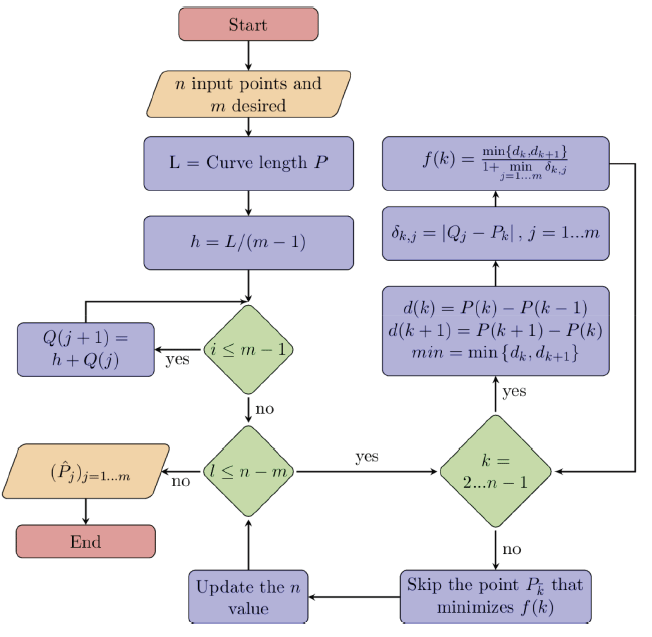Considering ${d}_{k}={P}_{k}-{P}_{k-1}$ and ${\delta }_{k,j}=|{Q}_{j}-{P}_{k}|$, note that for each Pk there is an ideal point Qj, such that δk,jh/2. In order to discard a point from the set ${\left({P}_{k}\right)}_{k=1, \dots ,n}$, we established an elimination rule, which seeks to eliminate the point such that k minimizes the following function:

$f\left(k\right)=\frac{\text{min}\left\{{d}_{k},{d}_{k+1}\right\}}{1+\underset{j=1\dots m}{\text{min}}{\delta }_{k,j}} k=2, \dots ,n-1$
(3)

The process above is applied (nm) times. In each iteration, the values of dk change due to the elimination rule of points in the set ${\left({P}_{k}\right)}_{k=1, \dots ,n}$ given by (3). Finally, we denote by ${\left({\stackrel{^}{P}}_{j}\right)}_{j=1, \dots ,m}$ the subset of points selected along the AdRe boundary. We implemented the algorithm described above in a Matlab function. Fig. 3 presents the result obtained when applying this algorithm in the sampling of the AdRe boundary of asteroid (1738) Oosterhoff.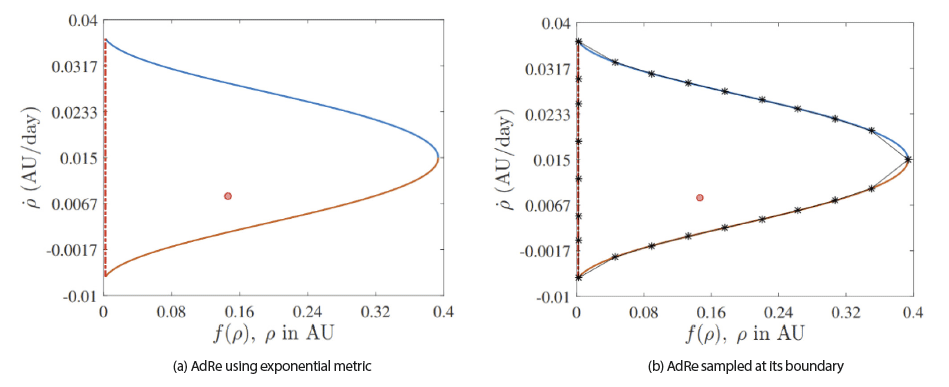Fig. 3. Sampling of boundary of the AdRe for asteroid (1738) Oosterhoff. The red dot shows the real position of the asteroid obtained from the HORIZONS Web-Interface service of NASA's JPL. AdRe, admissible region.
3.2 Internal Sampling of the AdRe

After the sampling of the AdRe boundary, it is necessary to sample the internal region. (Milani et al. 2004), and (Milani & Gronchi 2009) claim that an optimum method for improving this task consists of performing a triangulation followed by mesh smoothing. We propose a strategy based on the constrained Delaunay triangulation, which operates as follows. Given the domain of a polynomial $\stackrel{˜}{Ɗ}\subset Ɗ$ defined by the connection with the edges of the sample of boundary points (obtained in subsection 3.1) of the AdRe Ɗ, the triangulation of the polygonal domain $\stackrel{˜}{Ɗ}$ is the pair (Π,τ) with Π = {P1,...,PN} as the set of nodes of the domain, and τ = {T1,...,T2} as the set of triangles Ti, whose vertexes are in Π. This triangulation has to fulfill the following conditions:

• 1. ∪i = 1,k Ti = $\stackrel{˜}{Ɗ}$.

• 2. For each ij, the set TiTj is empty, or a vertex is empty, or a side of the triangle, or one of its edges.

If in addition to the set of points ∏, some edges PiPj are entered as inputs (for example, the boundary edges of $\stackrel{˜}{Ɗ}$; The input is referred to as a planar straight line graph [PSLG]), the triangulation that contains the prescribed edges is called a constrained triangulation.

For each triangulation (∏,τ), we can associate the minimum angle, which is defined as the minimum between the angles of all the triangles Ti. Among other possible triangulations of a convex domain, there is a construction called the Delaunay triangulation (Bern & Eppstein 1995), which is characterized by the following properties:

• ⅰ. It maximizes the minimum angle.

• ⅱ. It minimizes the circumscribed circle.

• ⅲ. For each triangle Ti, the internal part of its circumscribed circumference does not contain any node of the triangulation (Risler 1992).

When they are convex domains, the previous properties are equivalent. If the domain is a convex quadrangle whose vertexes ∏ are not on the same circle, there are two possible triangulations (∏,τ1), (∏,τ2). According to property (iii), only one of this triangulation is a Delaunay triangulation. In this case, the Delaunay triangulation is obtained through a first triangulation using a technique called edge-flipping (Milani & Gronchi 2009). Note that the AdRe domain, $\stackrel{˜}{Ɗ}$ is not generally convex. In this scenario, there is still a triangulation that maximizes the minimum angle, known as the constrained Delaunay triangulation, but the property (iii) is not guaranteed. For each triangle Ti A procedure is iterated over the adjacent triangles. If the common edge with the adjacent triangle is not Delaunay, the edge-flipping technique is applied. This procedure is repeated until all edges of the structure in the triangulation are Delaunay or are the edges of the boundary $\stackrel{˜}{Ɗ}$. In each repetition, the minimum angle increases, and the triangulation that maximizes the minimum angle is obtained at the end (Delaunay 1934).

In order to implement the triangulation described in the previous paragraphs, we develop an algorithm that begins by generating a constrained Delaunay triangulation (0,τ0) with the boundary points (generated as shown in 3.1) and the boundary edges. Once the initial triangulation is obtained, it is refined by adding internal points to the domain, maintaining the Delaunay property at each intersection. In each case, a new point is added, which extends to the internal part of the discrete density domain defined in the boundary point for the quantities:

$\rho {P}_{j}=\underset{l\ne j}{\text{min}}|{P}_{l}-{P}_{j}|,$

With the corresponding densities

$\stackrel{˜}{\rho }{G}_{i}=\frac{1}{3}\sum _{m=1}^{3}\rho {P}_{im},$

Where Gi corresponds to the barycenters of the triangles Ti and the barycenter ${G}_{\overline{k}}$ is added as a new point, which maximizes the minimum distance (weighted by its density $\stackrel{˜}{\rho }{G}_{\overline{k}}$ ) from the triangulation nodes (Pim, m = 1,...,3, belong to the same triangle Ti). Then, the corresponding triangle Tk is removed, and the triangles obtained by joining the edges of Tk with the new point are added to τ. Consequently, the optimum property of the Delaunay triangulation is conserved in each insertion.

The flow diagram in Fig. 4 shows the procedure used for triangulating the AdRe as a domain, which is generally not convex. It begins with a number of points resulting from the sampling of the boundary (for example, N = 25), out of which the repeated data are removed (the result of sampling the curves at its points of intersection). Then, a first triangulation is performed to identify the triangles outside the AdRe. Then, the domain is constrained, and a first constrained Delaunay triangulation is performed (the outer triangles are removed again).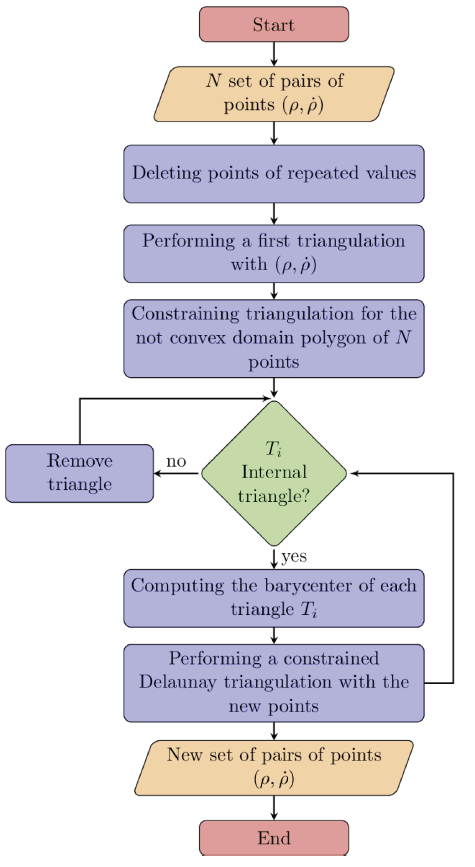Fig. 4. Constrained Delaunay triangulation algorithm for sampling inside the boundary of AdRe.

The following step consists of calculating the barycenters of each triangle Ti and with them performing a constrained Delaunay triangulation again. This last step is performed twice.

The algorithm in question was implemented as a MATLAB function. Fig. 5 presents the results obtained when applying our algorithm for sampling inside the AdRe boundary of asteroid (1738) Oosterhoff.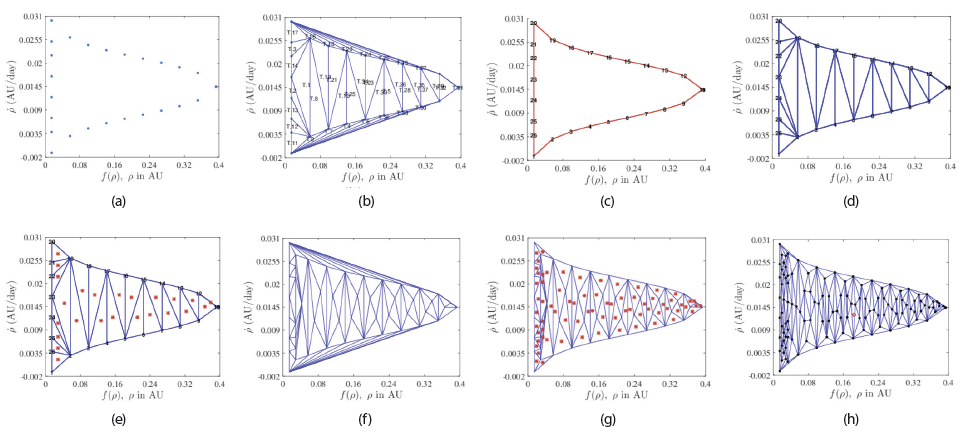Fig. 5. Results of applying our triangulation algorithm for sampling inside the AdRe boundary for asteroid (1738) Oosterhoff under the exponential metric. (a) Selection of points on the boundary. (b) First triangulation and identification of outer triangles. (c) Constraint of the AdRe domain. (d) Removal of triangles outside the domain. (e) First computation of barycenters. (f) Triangulation with the new set of points and subsequent constraint of the boundary. (g) New computation of barycenters inside the boundary of the AdRe. (h) Final result of both the boundary sampling process and constrained Delaunay triangulation. The red dot in (h) shows the asteroid real position. AdRe, admissible region.
3.3 Propagation of Orbits and Ephemeris Region

Once the boundary and the internal region of the AdRe are sampled, it is necessary to propagate the established points to define the possible orbits that belong to the object under study. In fact, this object corresponds to a minor body $\mathcal{\text{B}}$ that belongs to the solar system and that moves around the sun with heliocentric position r, which is observed from Earth ε with a radius vector R, known for a given instant of time. As is evident, the vector between the Earth and the minor body ρ is the unknown that was solved in the previous sections. This process gives, as a result, a set of possible values ($\rho ,\stackrel{˙}{\rho }$ ) for the average time of the observations collected from a TSA. Each of these points defines a virtual asteroid determined by a set of six quantities of the following form:

$X=\left[\alpha ,\delta ,\stackrel{˙}{\alpha },\stackrel{˙}{\delta },\rho ,\stackrel{˙}{\rho }\right]$

That set is known as attributable orbital elements (Milani & Gronchi 2009). The following step is consists of replacing each point ($\rho ,\stackrel{˙}{\rho }$ ) or the node of the triangulation (after going back to its original metric) in the state vector expression defined by equation (4).

$\begin{array}{c}r=R+\rho \stackrel{^}{\rho }\\ \stackrel{˙}{r}=\stackrel{˙}{R}+\stackrel{˙}{\rho }\stackrel{^}{\rho }+\rho \left(\stackrel{˙}{\alpha }{\stackrel{^}{\rho }}_{\alpha }+\stackrel{˙}{\delta }{\stackrel{^}{\rho }}_{\delta }\right)\end{array}$
(4)

Where $\stackrel{^}{\rho }$ is the unit vector in the direction of observation and $R,\stackrel{˙}{R}$ is the state vector of the Earth, a parameter that is obtained from the collection times of the TSA (Espitia Mosquera & Quintero Salazar 2019), with:

$\begin{array}{l}\stackrel{^}{\rho }=\left(\text{cos}\alpha \text{cos}\delta , \text{sin}\alpha \text{cos}\delta , \text{sin}\delta \right)\\ {\stackrel{^}{\rho }}_{\alpha }=\left(\text{-sin}\alpha \text{cos}\delta , \text{cos}\alpha \text{cos}\delta , 0\right)\\ {\stackrel{^}{\rho }}_{\delta }=\left(\text{-cos}\alpha \text{sin}\delta , -\text{sin}\alpha \text{sin}\delta , \text{cos}\delta \right)\end{array}$
(5)

As a result of this process, a set of initial state vectors ($r,\stackrel{˙}{r}$) in the heliocentric-equatorial system is obtained. We used a two-body integrator based on the functions f and g (Danby 1962;Boulet 1991) to obtain the set of final state vectors (rf,${\stackrel{˙}{r}}_{f}$). In order to simulate the propagation for a few days we use this dynamic model because its implementation is relatively simple to implement. Fig. 6 presents this set for the case of asteroid (1738) Oosterhoff. From this set, we calculate the ephemerides, that is, the right ascension and declination coordinates for each of the points propagated for a specific date after the observation of the object under study (ephemerides region).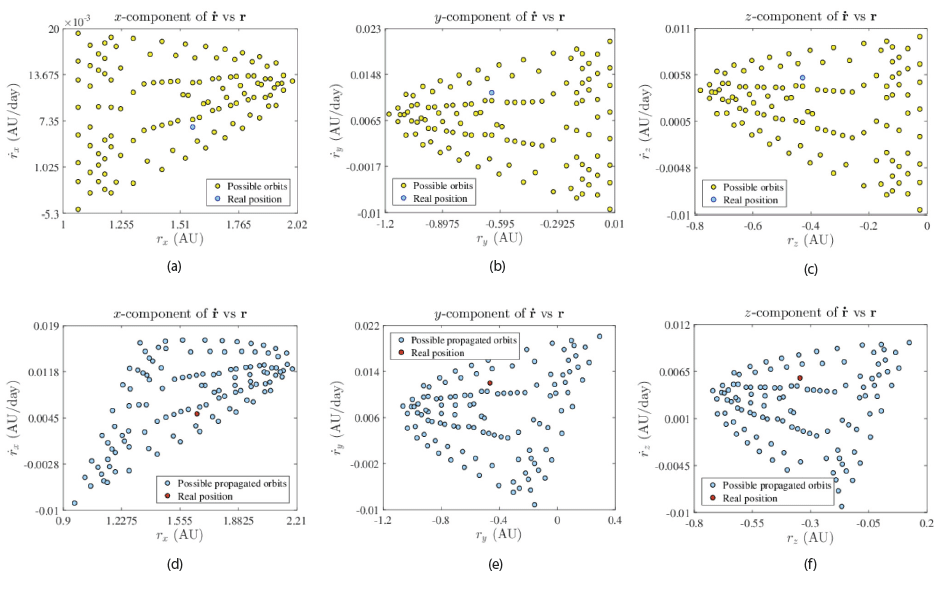Fig. 6. Components of heliocentric position and velocity vectors for asteroid (1738) Oosterhoff. Top row: vectors at the average time of initial observation. Bottom row: vectors at the recovery time. The cyan and red dots show the components of the real asteroid.

## 4. RESULTS

We applied our follow-up strategy to the set of the 12 asteroids listed in the first column of Table 1, whose types are reported in the fourth column (6 NEA, 2 MBA, 1 Hungaria, 2 Hilda, and 1 Jupiter trojan). In order to achieve this goal, we use our new web recovery service available at http://observatorioenlinea.utp.edu.co/recoveryservice/.

Table 1. Table of sample asteroids recovery. The observatory code, the interval of the initial observations, the orbit type, the Smax value, the proper motion, the propagated days interval and the possibility of a recovery are shownAs input data, we used the observations reported to the MPC (https://www.minorplanetcenter.net/db_search) by the observatories listed in the second column within the intervals of observation presented in the third column (in all cases, the interval of observation used constitutes a TSA). For minor bodies reported by the W63 observatory, they are registries performed by us from the Astronomical Observatory of the Technological University of Pereira, Colombia (from here on, OAUTP) exclusively for this work. The complete tables of data of the observations used in this work are available at https://www.utp.edu.co/ observatorioastronomico/astrometria/recovery.

First, we established the AdRe's for the minor bodies under study following the procedure described in Espitia Mosquera & Quintero Salazar (2019), using the geocentric and topocentric approximations and the exponential and logarithmic metrics.

The performed tests showed that for the 12 asteroids analyzed, the most adequate version for performing an optimum sampling process was the version with topocentric correction and based on the exponential metric. This is because the topocentric approximation gives, in all cases, AdRe's with much simpler shapes than their geocentric equivalents, and the exponential metric presents a higher density of points around the asteroid’s real position. Figs. 7 and 8 present the AdRe's computed for the objects under study. However, we observed that the logarithmic metric describes regions of the AdRe's near the Earth with more detail, so it would be useful in the study of near meteoroids and artificial satellites. For those readers interested in these types of objects, we report the AdRe's obtained using logarithmic metrics at https://www.utp.edu.co/ observatorioastronomico/astrometria/recovery.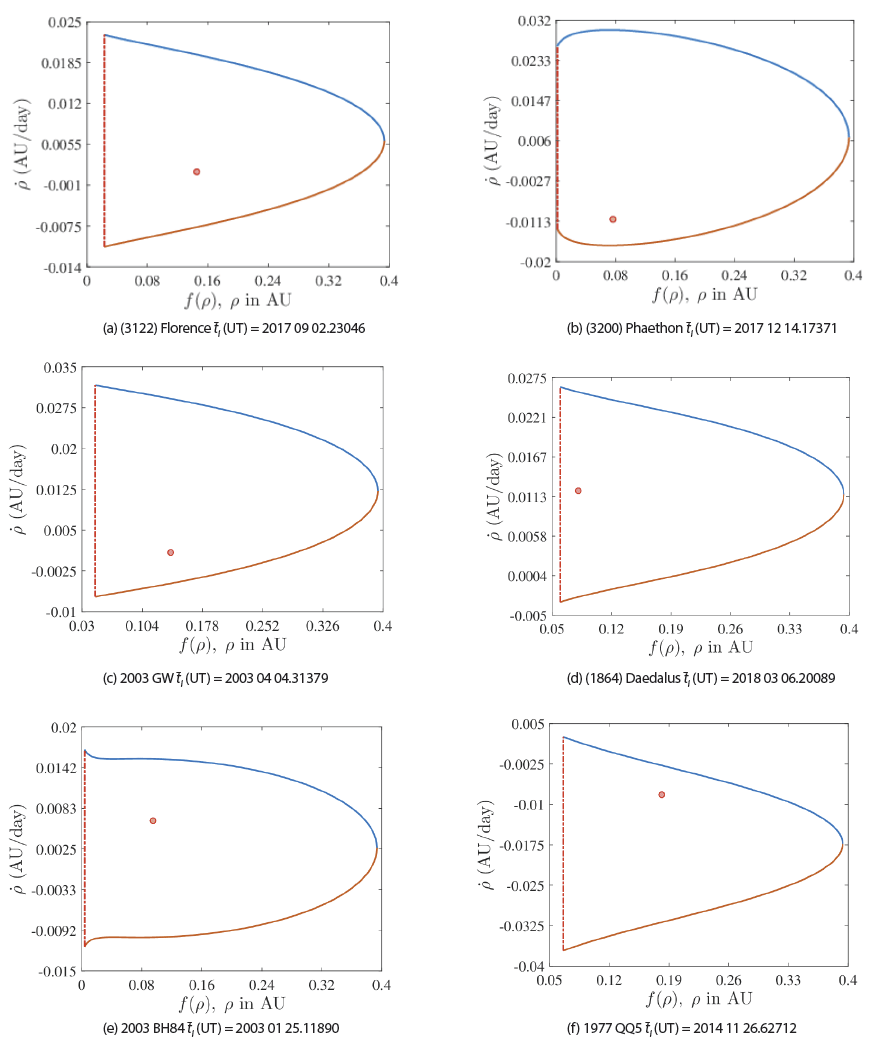Fig. 7. AdRe's for asteroids (3122) Florence, (3200) Phaethon, 2003 GW, (1864) Daedalus, 2003 BH84 and 1977 QQ5, with topocentric correction using the exponential metric. The red dot shows the real position of the asteroid obtained from the HORIZONS Web-Interface service of NASA's JPL.Fig. 8. AdRe's for asteroids (1738) Oosterhoff, (4690) Strasbourg, (555) Norma, 2006 SO375, 2003 GE55 and (32811) Apisaon, with topocentric correction using the exponential metric. The red dot shows the real position of the asteroid obtained from the HORIZONS. AdRe, admissible region.

Regarding the AdRe's calculated under the topocentric approximation and under exponential metrics, we applied the sampling technique described in section 3. Figs. 9 and 10 present the results of this process. In all cases, our sampling method generated at least one node of the triangulation in the proximities of the asteroid’s real position. This indicates that at least one of the ephemeris points corresponds to a value close to the asteroid’s real position in the celestial sphere.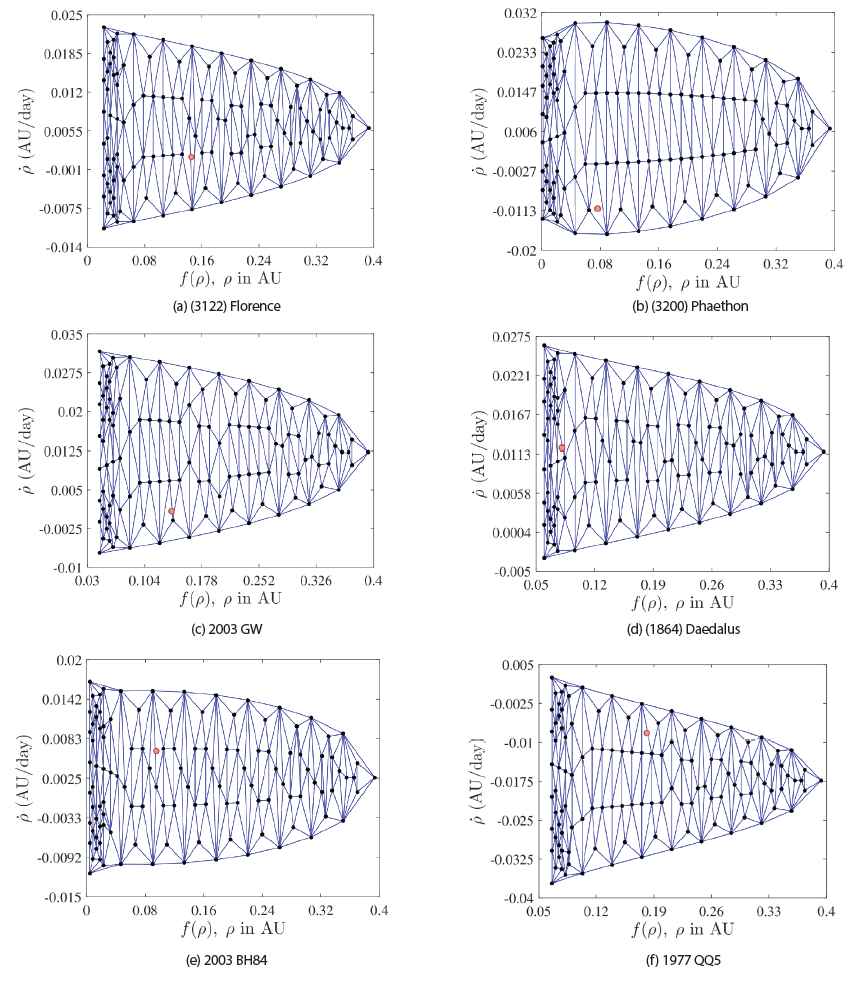Fig. 9. Triangulated AdRe's for asteroids (3122) Florence, (3200) Phaethon, 2003 GW, (1864) Daedalus, 2003 BH84 and 1977 QQ5. The red dot shows the real position of the asteroid obtained from the HORIZONS Web-Interface service of NASA's JPL. AdRe, admissible region.Fig. 10. Triangulated AdRe's for asteroids (1738) Oosterhoff, (4690) Strasbourg, (555) Norma, 2006 SO375, 2003 GE55 and (32811) Apisaon. The red dot shows the real position of the asteroid obtained from the HORIZONS Web-Interface service of NASA's JPL. AdRe, admissible region.

We transformed each node provided by the triangulation into a heliocentric-equatorial state vector ($\rho ,\stackrel{˙}{\rho }$). Then, we performed the calculation of the set of vectors for the position and velocity in the heliocentric-equatorial system (${r}_{i},{\stackrel{˙}{r}}_{i}$). The graphs of each component for the initial times of each asteroid show that the metric that offers a cleaner sampling was the exponential one (https:// observatorioastronomico.utp.edu.co/astrometria/recovery. html). Subsequently, we performed propagation of each of these vectors to a date after the observation (column 8, Table 1), the date at which the follow-up of the asteroids in question was performed. For that purpose, we used our 2-body propagation algorithm through functions f and g. The propagation of these vectors showed that there are deformations of the AdRe’s for the spatial components.

The most significant change occurred for asteroids (3200) Phaethon and (3122) Florence, whose input data were collected when they were at their points of closest approach to the Earth (the real position of the objects within their orbit at the moment of their observation is listed in column 7 of Table 1). The changes in the shape of the AdRe’s, after the propagation, become less abrupt when the asteroid is farther away from the observer.

Finally, we calculated the ephemerides for each of the points obtained from the previous process for observation times after the observations listed in column 8 of Table 1. The recovery times are calculated from the location of the OAUTP (W63). Figs. 11 and 12 present the results of this process. As a reference, the figures include, indicated within the blue dotted-line box, the field of the vision given by the instrumental assembly of the OAUTP (95’ × 72’). Our approach is to slew the telescope near the region with the highest density of points, i.e., the region with more ephemeris grouped. The asteroid’s real position is also included; it was obtained from the HORIZONS Web- Interface of NASA’s JPL (identified by a red dot; https:// ssd.jpl.nasa.gov/horizons.cgi) and the recovery by Väisälä provided by the New Object Ephemerides service of the MPC (identified by a blue dot; http://www.minorplanetcenter. net/iau/MPEph/NewObjEphems.html). We tested our methodology by implementing a Laplacian mesh smoothing filter. However, as our purpose was to point the telescope towards the region of the sky with the highest number of points (i.e., ephemeris), the difference when we applied the filter did not show a more significant advantage in the results shown in Figs. 11 and 12. So we concluded that our method eliminates the need for smoothing as proposed by Milani et al. (2004), thus increasing the computational efficiency.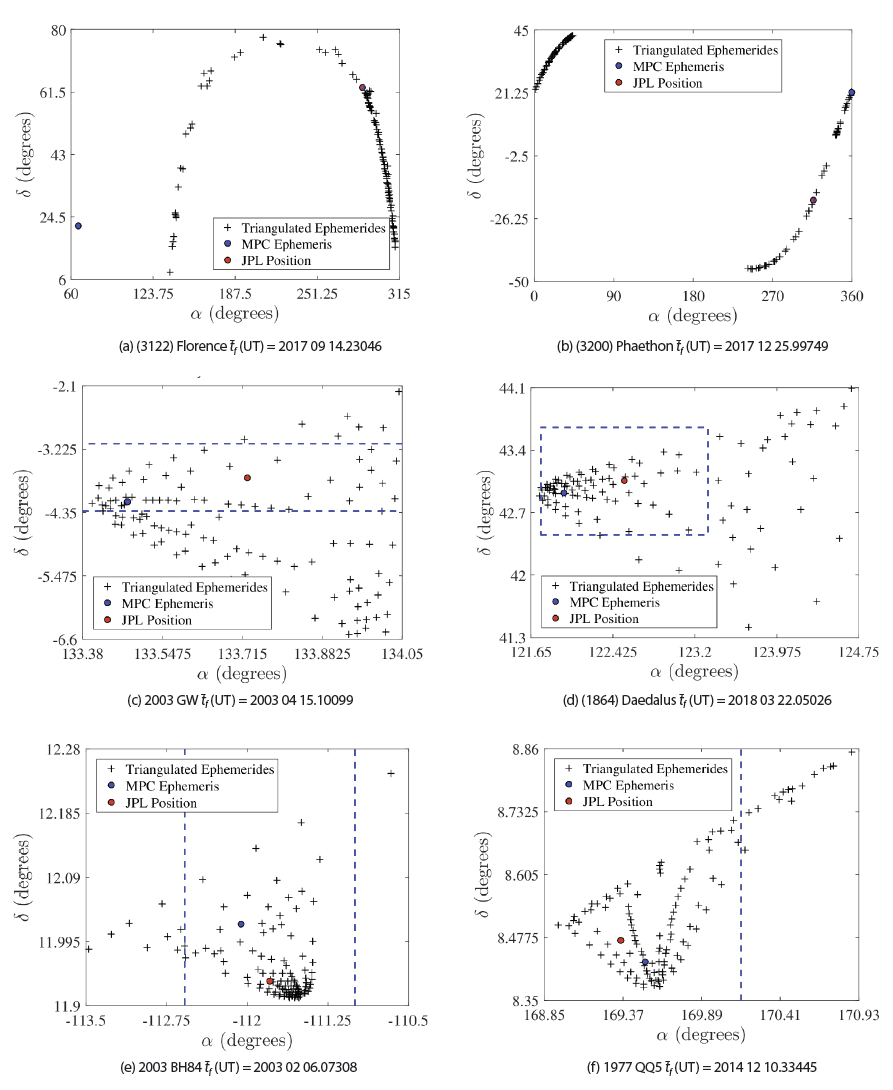Fig. 11. Ephemerides region for final date tf for asteroids (3122) Florence, (3200) Phaethon, 2003 GW, (1864) Daedalus, 2003 BH84 and 1977 QQ5. The blue dotted lines box corresponds to the field of vision (95' × 72') given by the OAUTP instrumental assembly.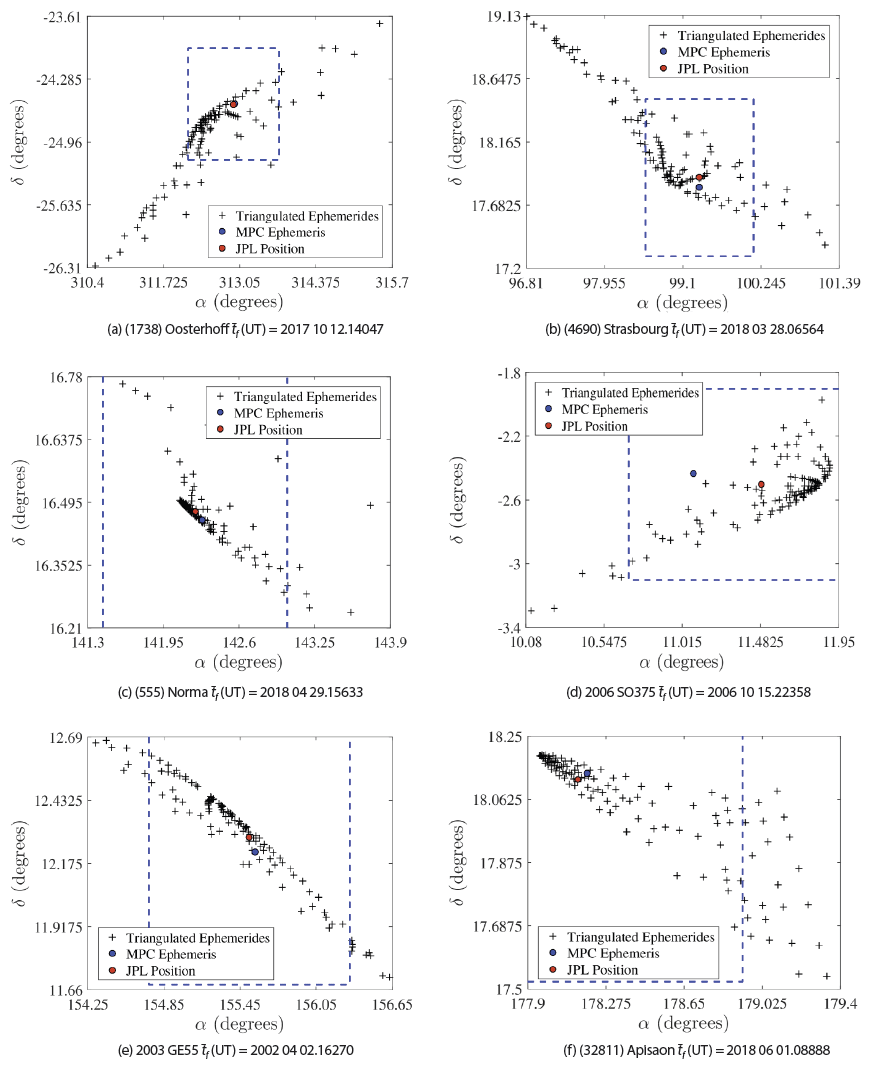Fig. 12. Ephemerides region for final date tf for asteroids (1738) Oosterhoff, (4690) Strasbourg, (555) Norma, 2006 SO375, 2003 GE55 and (32811) Apisaon. The box comprised of blue dotted lines corresponds to the field of vision (95' × 72') given by the OAUTP instrumental assembly.

As can be observed from Figs. 11 and 12, except for asteroids (3122) Florence and (3200) Phaethon, all asteroids were recoverable since they presented a higher density of possible location coordinates within the visual field of the OAUTP and near the asteroids’ real position (red dot). In some cases, it is sufficient to recover the object again by panning the sky with a telescope that covers three fields of vision. Even in the cases of asteroids 2003 BH84 and 2006 SO375, our recovery was better than that provided by the New Object Ephemerides service since our triangulated ephemerides have a higher density closer to the real position than that given by the MPC. In addition, column 8 of Table 1 shows that the follow-up times range from 10 days (in the case of asteroid 2003 GW) up to 47 days (for the case of asteroid 2003 GE55) after the observations, from intervals of observation that do not exceed 3 hours, which demonstrates the potential of our follow-up method of newly discovered objects. Our method also reduces the number of objects that inflate the lists of lost objects, since the follow-up strategy would enable locating an object up to 47 days after their discovery, using an interval of the observation of just 3 hours.

In the case of asteroids (3122) Florence and (3200) Phaethon, our method was not able to anticipate a recovery (last column of Table 1). In fact, the New Object Ephemerides service of the MPC was not able to either (see the top row of Fig. 11). This was because the input observations correspond to one of the moments of closest approach to the Earth (in this case, of the order of 10–2 au), and therefore their apparent motion was large (column 6 of Table 1). In the recording of a close pass by the Earth, the gravitational field can affect our propagation model based on a two-body integrator, so we conclude that in these cases, it is necessary to consider the gravity of the Earth as a perturbation in our dynamical model.

In order to identify the scope of our method, we repeat the follow-up of asteroid 2006 SO375, but now with just two input data separated by less than 2 minutes, corresponding to the night of their discovery (Table 2). Fig. 13 presents the ephemeris region of the asteroid for 28 days after its observation. Note how the higher density of possible positions of the asteroid provided by our algorithm (black crosses) is within the field of the vision given by the instrumental assembly of the OAUTP and closer to the real position given by the JPL’s HORIZONS Web- Interface (red dot), than the recovery provided by the New Object Ephemerides service of the MPC (blue dot). This demonstrates the scopes of our technique, in addition to its application in the follow-up of recently discovered minor bodies.

Table 2. Table of recovery for asteroid 2006 SO375 using an interval of Δt = 1 min 11.712 s. The initial date of observation, the recovery final date, the proper motion and the Smax value are shown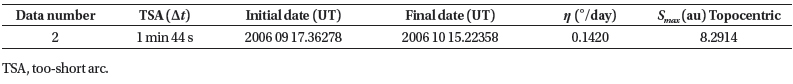Fig. 13. Ephemerides region for asteroid 2006 SO375 from 2 spaced data Δt = 1 min 11.712 s.

## 5. DISCUSSION AND CONCLUSION

Literature review showed that the AdRe sampling technique is an important tool for the study of objects (especially with short intervals of observation) that is widely applied to space debris. However, despite its versatility, we found in our review that the use of AdRe has only been applied for the following minor bodies in two cases: 2003 BH84 and (60558) Echeclus. In this paper, we extend the field of application of the AdRe sampling followup technique by applying it to a group of 12 asteroids: 6 NEAs and 6 MBAs. Regarding the sampling strategy, we proposed a technique based on the constrained Delaunay triangulation, which does not require the subsequent application of a mesh smoother. In addition, we implement our follow-up algorithm in a web service under an opensource license. We constructed the AdRe's for the twelve asteroids from the geocentric approximation and, with topocentric correction, implementing the logarithmic and exponential metrics.

The results showed that the AdRe's based on topocentric correction and using the exponential metric were better adapted to our sampling technique, since they presented a greater geometric simplicity and a higher density of possible orbits around the asteroids’ real position. In addition, we observed that the AdRe's presented common elements within each family of asteroids, such that they could be used in future work to delimit the possible families to which a newly discovered object belongs.

For the 12 asteroids, we propagated the ephemeris region using the field of vision of the OAUTP (W63). We observed that 10 out of the 12 asteroids were recoverable, with observation times between approximately 25 minutes and 2 hours (TSA), and with propagations for follow-up of up to 47 days. We even observed how with an interval of the observation of just 2 minutes, it was possible to recover asteroid 2006 SO375 for approximately 28 days, which demonstrates the potential of our triangulation technique. The fact that our methodology can perform the follow-up of an object, even several weeks after its observation, makes it possible for observatories exposed to changing climate conditions, as in the case of the OAUTP, to observe the object again. This enables gathering more data, which would make it possible to construct a preliminary orbit, avoiding addition of the object to the list of lost objects. In the case of asteroids (3122) Florence and (3200) Phaethon, we could not perform the recovery from the observations used as input. We concluded that this was due to these objects being at the nearest point to Earth within their orbit at the moment of observation. As can be observed from Table 1, these minor bodies had a position ρ of the order 10–1 au and an average motion η much faster than the other asteroids. To validate our hypothesis, we simulated observations for this same pair of objects but at a distance greater than 1 au. In this case, our method did allow the follow-up of the objects. The follow-up that we obtained for asteroid 2003 GW (ρ ≌ 1 au) allows us to infer that the closer the object under study is from the Earth at the moment of observation, the more dispersed will be its ephemeris region (centerleft, Fig. 11). This effect could be due to its proximity to the Earth, explained by the gravitational field has a more drastic influence, which is why it would be necessary to implement a three-body model in this type of case.

Currently, there are two web services JPL's Scout system (https://cneos.jpl.nasa.gov/scout) and NEOScan (https:// newton.spacedys.com/neodys/NEOScan/), which allow obtaining ephemeris from short-arcs observation by using sophisticated methods. However, both services have a common disadvantage which is the fact that they only allow calculating ephemeris of existing objects in the Near-Earth Object Confirmation Page database (https:// minorplanetcenter.net//iau/NEO/toconfirm_tabular.html). In contrast, our services allow obtaining ephemeris from the coordinates resulting from the astrometric reduction made directly by the observer. In this way, if an observed object is suspected of being a discovery, the observer has the possibility of planning its future observations using our service without the need to wait for the provisional pre-registration of the object in the Near-Earth Object Confirmation database.

## ACKNOWLEDGMENTS

We are grateful for financial support provided by the “Vicerrectoría de Investigaciones, Innovacióny Extensión” of the Universidad Tecnológica de Pereira, Colombia. This research has made use of NASA's Astrophysics Data System Bibliographic Services.

## References

1.

Bern N, Eppstein D, Mesh generation and optimal triangulation, in Computing in Euclidean Geometry, eds. Du D, Hwang F (World Scientific, Singapore, 1995), 47-123.2.

Boulet DL, Methods of Orbit Determination for the Microcomputer (Willmann-Bell, Richmond, NA, 1991).3.

Danby DMA, Fundamentals of Celestial Mechanics (Macmillan, London, 1962).4.

Delaunay B, Sur la sphere vide, Izv. Akad. Nauk SSSR, Otdelenie Matematicheskii i Estestvennyka Nauk 7, 6, 793-800 (1934).5.

DeMars KJ, Jah MK, Schumacher PW, Initial orbit determination using short-arc angle and angle rate data, IEEE Trans. Aerosp. Electron. Syst. 48, 2628-2637 (2012).6.

Espitia D, Quintero EA, Arellano-Ramirez ID, Determination of orbital elements and ephemerides using the geocentric Laplace’s method, J. Astron. Space Sci. 37, 171-185 (2020).7.

Espitia Mosquera D, Quintero Salazar EA, Determination of the admissible region of asteroids with data from one night of observation, J. Phys. Conf. Ser. 1247, 012038 (2019).8.

Farnocchia D, Chesley SR, Milani A, Gronchi GF, Chodas PW, Orbits, Long-Term Predictions, and Impact Monitoring, Asteroids IV, eds. Michel P, DeMeo FE, Bottke WF (University of Arizona Press, Tucson, AZ, 2015), 815-834.9.

Farnocchia D, Tommei G, Milani A, Rossi A, Innovative methods of correlation and orbit determination for space debris, Celest. Mech. Dyn. Astron. 107, 169-185 (2010).10.

Gronchi GF, Classical and modern orbit determination for asteroids, Proc. Int. Astron. Union. 2004, 293-303 (2004).11.

Gwyn SDJ, Hill N, Kavelaars JJ, SSOS: a moving-object image search tool for asteroid precovery, Publ. Astron. Soc. Pac. 124, 579-585 (2012).12.

Kristensen LK, Initial linking methods and their classification, Proc. Int. Astron. Union. 2, 301-308 (2006).13.

Maruskin JM, Scheeres DJ, Alfriend KT, Correlation of optical observations of objects in Earth orbit, J. Guid. Control Dyn. 32, 194-209 (2009).14.

Milani A, The asteroid identification problem IV: attributions, Icarus. 151, 150-159 (2001).15.

Milani A, Gronchi G, Theory of Orbit Determination (Cambridge University Press, Cambridge, 2009).16.

Milani A, Gronchi GF, Vitturi MDM, Knežević Z, Orbit determination with very short arcs. I Admissible regions, Celest. Mech. Dyn. Astron. 90, 57-85 (2004).17.

Milani A, Knežević Z, From astrometry to celestial mechanics: orbit determination with very short arcs, Celest. Mech. Dyn. Astron. 92, 1-18 (2005).18.

Risler JJ, Mathematical Methods for CAD (Cambridge University Press, Cambridge, 1992).19.

Spoto F, Del Vigna A, Milani A, Tommei G, Tanga P, et al., Short arc orbit determination and imminent impactors in the Gaia era, Astron. Astrophys. 614, A27 (2018).20.

Tommei G, Milani A, Rossi A, Orbit determination of space debris: admissible regions, Celest. Mech. Dyn. Astron. 97, 289-304 (2007).21.

Väisälä Y, Eine einfache Methode der Bahnbestimmung (Suomalaisen Tiedeakatemian Kustantama, Helsinki, 1939).22.

Valk S, Lemaitre A, Admissible regions for too short arcs: nodal distances and elongations, Proceedings of the International Astronomical Union, Prague, 24 Aug 2006.